Results as of FPCP 2006

Menu:

 Δms results Constraint from BR(B+→τ+ ν) [with new Belle result] The Global CKM fit: Inputs and Numerical results The global CKM fit in the large (ρ-bar,η-bar) plane The global CKM fit in the small (ρ-bar,η-bar) plane (zoom) Constraints on the angle α/Φ2 from charmless B decays Constraints on the angle γ/Φ3 from B decays to charm Constraints on |sin(2β+γ)|

Numerical results:

 A brief document that provides the collection of up-to-date inputs to the global CKM analysis and the numerical results obtained from it. The results include: Wolfenstein parameters, UT angles, (combinations of) CKM elements, theory parameters and rare branching fractions. Detailed background information on the methodology and the treatment of experimental and theoretical uncertainties is provided in hep-ph/0406184.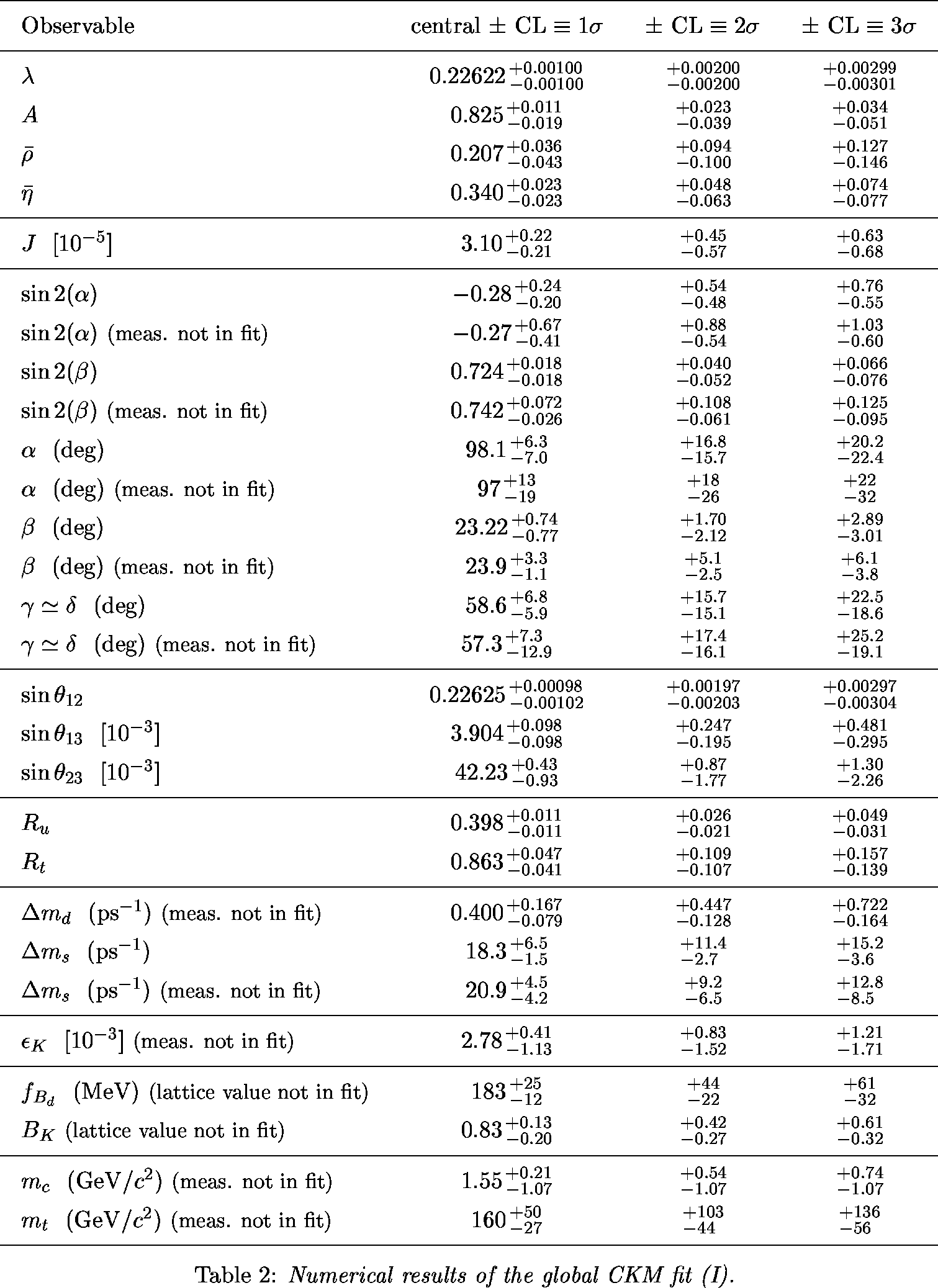ps pdf

ΔMs Results

 α,β,γ convention: Constraints in the (ρ-bar,η-bar) plane with FPCP06 inputs and experimental measurements except ΔMs as of Summer 2005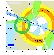eps gif Constraints in the (ρ-bar,η-bar) plane with FPCP06 inputs and experimental measurements except ΔMs as of Moriond 2006 (with D0 result but not CDF result).eps gif Constraints in the (ρ-bar,η-bar) plane with FPCP06 inputs and experimental measurements (with D0 and CDF ΔMs results)eps gif Comparison between CDF ΔMs measurement and CKM fit w/o ΔMs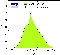eps gif |Vtd/Vts| from the global fit, the CDF ΔMs measurement and the ratio of the branching fractions (B→ρ0γ / B→K*0 γ) (average of BABAR and Belle)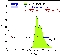eps gif

 α,β,γ convention: φ1,φ2,φ3 convention: Constraint in the (ρ-bar,η-bar) plane from the simultaneous use of the the B+→τ+ν branching fraction and Δmd.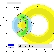eps gifeps gif Constraint in the (ρ-bar,η-bar) plane from the simultaneous use of the the B+→τ+ν branching fraction and Δmd with improved staggered fermions (see hep-lat/0510113).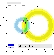eps gif Constraint in the (ρ-bar,η-bar) plane from the simultaneous use of the limit on the B+→τ+ν branching fraction and Δmd. Also shown is the constraint one would obtain by a precise measurement of BR(B+→τ+ν). It illustrates the gain in accuracy obtained from the simultaneous use of the two constraints ( B+→τ+ν and Δmd), where the uncertainty from fB is reduced (it cancels in the ratio).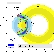eps gifeps gif

 α,β,γ convention: φ1,φ2,φ3 convention: Constraints in the (ρ-bar,η-bar) plane including (a.o.) the most recent α/Φ2- and γ/Φ3-related inputs in the global CKM fit.eps gifeps gif Constraints in the (ρ-bar,η-bar) plane not including the angle measurements in the global fit.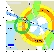eps gifeps gif Constraints in the (ρ-bar,η-bar) plane not including the angle measurements.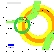eps gifeps gif Constraints in the (ρ-bar,η-bar) plane including only the angle measurements.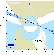eps gifeps gif Constraints from CP conserving quantities (|Vub / Vcb|, Δmd, (Δmd and Δms) and B+ →τ+ ν) in the (ρ-bar,η-bar) plane.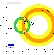eps gifeps gif Constraints from CP violating quantities (sin(2β), α, γ and εk) in the (ρ-bar,η-bar) plane.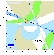eps gifeps gif

 α,β,γ convention: φ1,φ2,φ3 convention: Zoomed constraints in the (ρ-bar,η-bar) plane including the most recent α/Φ2- and γ/Φ3-related inputs in the global CKM fit.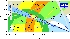eps gifeps gif Zoomed constraints in the (ρ-bar,η-bar) plane not including the angle measurements in the global fit.eps gifeps gif Zoomed constraints in the (ρ-bar,η-bar) plane not including the angle measurements but sin2β.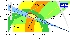eps gifeps gif Zoomed constraints in the (ρ-bar,η-bar) plane not including the angle measurements but sin2β and α.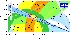eps gifeps gif Zoomed constraints in the (ρ-bar,η-bar) plane not including the angle measurements.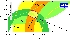eps gifeps gif Constraints in the (ρ-bar,η-bar) plane including only the angle measurements.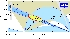eps gifeps gif Constraints from CP conserving quantities (|Vub / Vcb|, Δmd, (Δmd and Δms) and B+ →τ+ ν) in the (ρ-bar,η-bar) plane.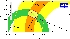eps gifeps gif Constraints from CP violating quantities (sin(2β), α, γ and εk) in the (ρ-bar,η-bar) plane.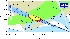eps gifeps gif Constraints from "Tree" quantities in the (ρ-bar,η-bar) plane.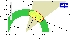eps gifeps gif Constraints from "Loop" quantities in the (ρ-bar,η-bar) plane.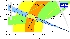eps gifeps gif

 Constraint on α/Φ2 from B→ρρ.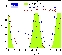eps gif Constraints on α/Φ2 from B→ππ, ρπ, ρρ, compared to the prediction from the CKM fit (not including these measurements). α[combined] = 100.2 +15.0/–8.8 deg.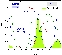eps gif The numerical results for these quantities are given in the summary paper

 Constraints on γ/Φ3 from world average D(*)K decays (GLW+ADS) and Dalitz analyses compared to the prediction from the global CKM fit (not including these measurements). γ[combined] = 62 +35/–25 deg.eps gif The numerical results for these quantities are given in the summary paper

 Constraints on |sin(2β+γ)| from the measurement of time-dependent CP asymmetries in D(*) π (ρ). Moriond06 HFAG average is used as input. The extraction of the UT angles relies on SU(3) symmetry for the estimates of the suppressed-to-leading amplitude ratios. We use r = 0.013 ± 0.0023 and r* = 0.019 ± 0.0029, and apply an additional theoretical uncertainty in form of a 15% error range to these.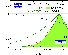eps gif Translation of this result into γ (using sin(2β) as additional input and choosing among the four solutions to the SM one). γ[GLW+ADS+GGSZ+|sin(2β+γ)|] = 71 +22 / – 30°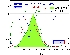eps gif Constraints from |sin(2β+γ)| in the (ρ-bar,η-bar) plane.eps gif